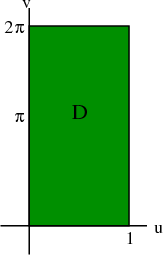# Math Insight

### Image: Rectangular domain to be mapped into helicoidThe region $\dlr$ described by $0 \le \spfv \le 1$ and $0 \le \spsv \le 2\pi$ is mapped by the function $\dlsp(\spfv,\spsv) = (\spfv\cos \spsv, \spfv\sin \spsv, \spsv)$ into a helicoid.

Image file: rectangle_helicoid_domain.png